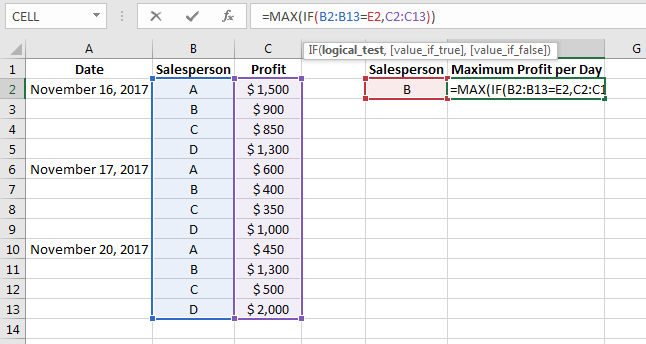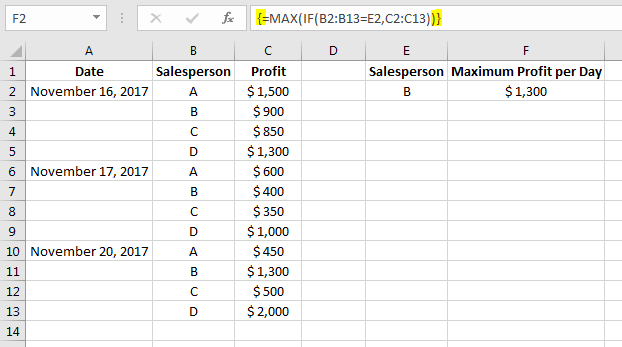# Excel Max and If

### Max and If Functions

This is one of the most useful trick in Excel. The tutorial will demonstrate how to get the maximum value given an array and condition.

Description of Example
The objective is to find the maximum profit per day of salesperson B.

Evaluation of Formula
The IF function filters the array at column B to match the value at cell E2, it then populates the data at column C when it matches the criteria "Salesperson B". The maximum value are then returned out from the filtered data using MAX function.WATCH IT NOTES
This is an array formula, and for it to work press Ctrl + Shift + Enter at the end of the function. Brackets "{ }" will automatically appear to enclosed the function, indicating an array.